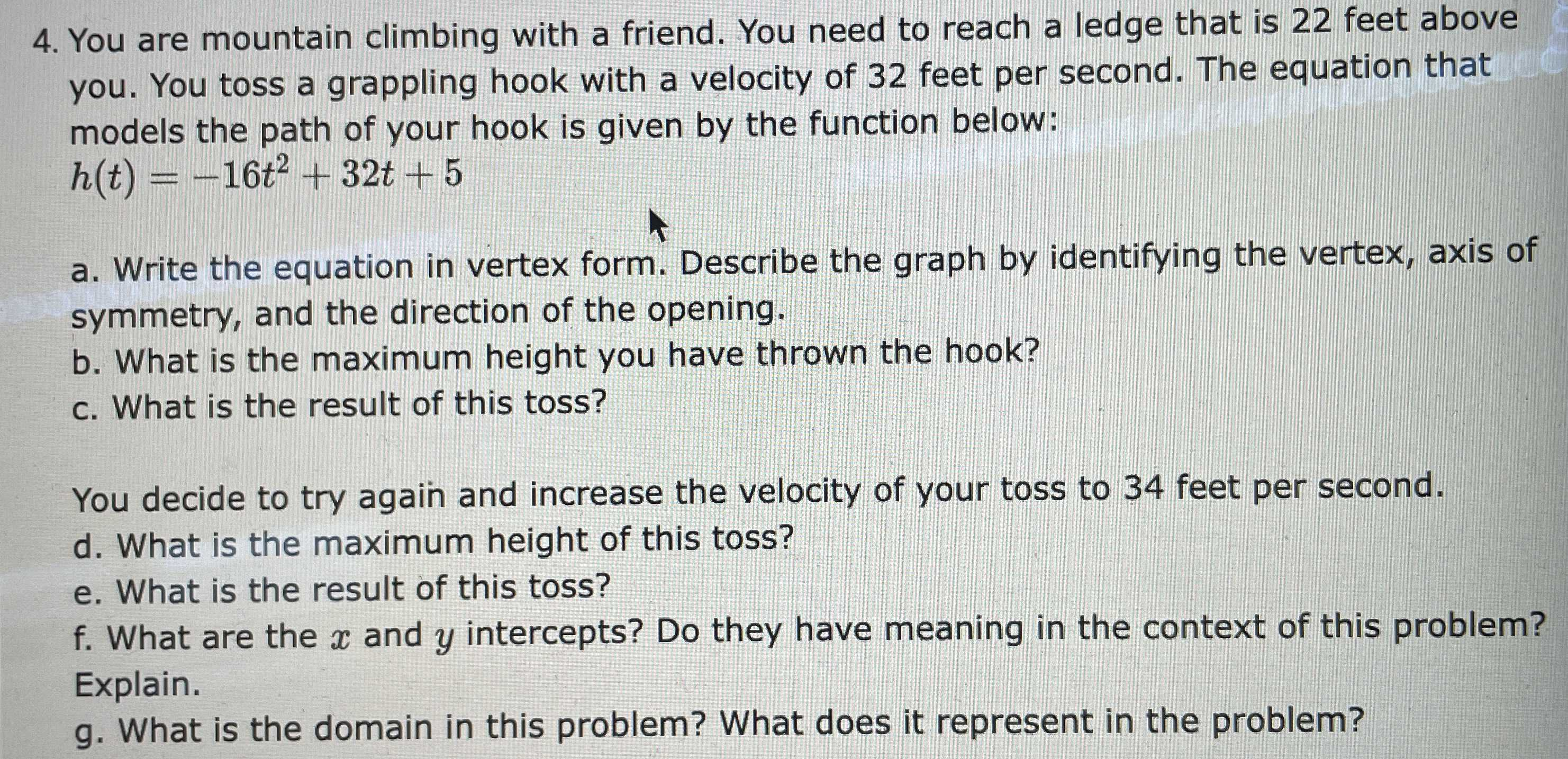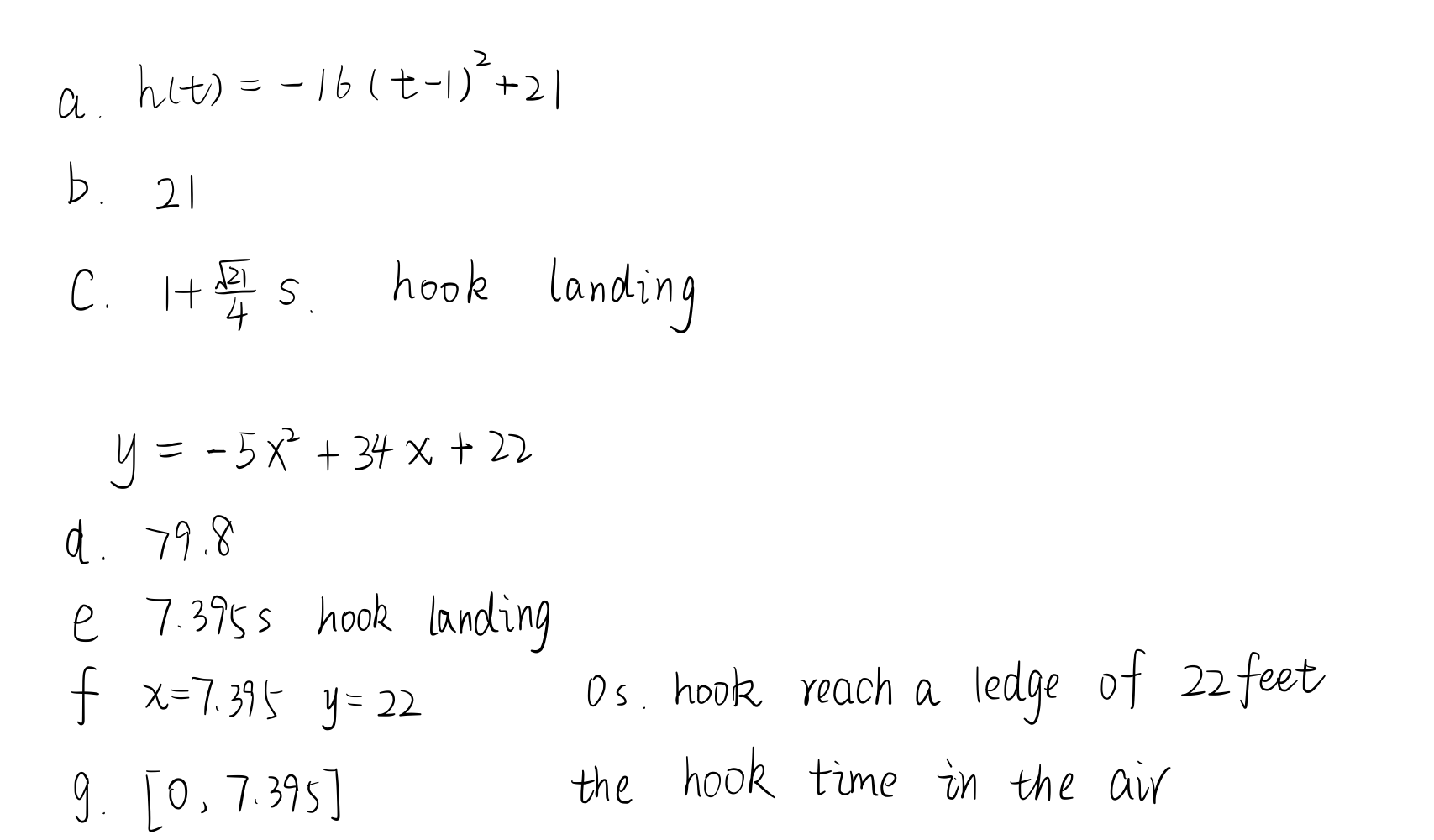### ¿Todavía tienes preguntas de matemáticas?

Pregunte a nuestros tutores expertos
Algebra
Pregunta4. You are mountain climbing with a friend. You need to reach a ledge that is $$22$$ feet above you. You toss a grappling hook with a velocity of $$32$$ feet per second. The equation that models the path of your hook is given by the function below:

$$h ( t ) = - 16 t ^ { 2 } + 32 t + 5$$

a. Write the equation in vertex form. Describe the graph by identifying the vertex, axis of symmetry, and the direction of the opening. b. What is the maximum height you have thrown the hook? c. What is the result of this toss? You decide to try again and increase the velocity of your toss to $$34$$ feet per second. d. What is the maximum height of this toss? e. What is the result of this toss? f. What are the $$x$$ and $$y$$ intercepts? Do they have meaning in the context of this problem? Explain. g. What is the domain in this problem? What does it represent in the problem?